# Estimating Expectations with Samples

The problem of computing an expectation of a function that takes a random variable as an input crops up in many areas of statistics and machine learning. There are numerous ways of estimating this expectation such as by using Markov Chain Monte Carlo (MCMC) methods or by using variational inference. In this short read, I’ll discuss the idea of using samples to estimate expectations and introduce one such MCMC method — Gibbs sampling — and how it can be used to estimate these expectations. But first, a bit on notation…

We say samples of a random variable x is drawn from a distribution P(X) as x ~ P(x). X is the sample space/domain and x is a random variable and an instance from this sample space. For example, let’s imagine we have an unbiased coin such that it has equal probability of showing heads and tails during a toss. In this case, the sample space, X = {heads, tails}. The probability that x = heads is P(x = heads) = 0.5.

Let’s say, we have a continuous random variable drawn from a unit normal distribution, ie, x ~ N(0, 1), where the mean μ = 0 and the variance σ = 1. Drawing samples from a unit normal distribution is relatively simple and can be easily done in numpy with a simple function call:

Well, that was easy! How about drawing a sample from not a unit normal but a normal distribution with a specific mean μ = 10 and σ = 3?

That is not difficult to do either. We can easily transform a sample, x, drawn from a unit normal to a sample, y, drawn from N(μ, σ) using the transformation: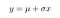Samples of y which is drawn from a particular normal distribution with mean and variance can be drawn by first drawing samples of x from a unit normal and then transforming the samples.

The examples we’ve seen so far might give the illusion that it is easy to sample from an arbitrary probability density function P. It is easy to sample from distributions such as the normal or the uniform distribution but that’s absolutely not true for any arbitrary distribution P!

The complexity of sampling from an arbitrary distribution comes from the fact that probability distributions over the entire domain (sample space) must sum to 1. Oftentimes what we have access to is the unnormalised density function, T. To obtain a valid distribution P from the unnormalised densities, we need to divide values of T(x) over the entire domain of x, (the domain of x is denoted by X — note the capitalization) by the normalizing factor, Z: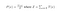T is the unnormalized density. T is provided over the entire domain of x. To get a probability distribution over x, the values of T(x) need to be divided by the normalizing factor Z, which ensures P is a valid distribution and sums to 1.

For high-dimensional values of x, computing the normalizing factor becomes intractable because T(x) will need to be evaluated over the entire domain X. More concretely, to compute Z for an n-dimensional samples of x from X: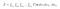Computing the normalizing constant, Z, is hard, especially when the random variable, x, is high dimensional. This is because when x is high dimensional, to compute the normalizing constant Z, we need to integrate over the entire domain of each dimension of x, which is intractable.

# Why do we care about sampling from P ?

Let’s say we have a continuous random variable x and a deterministic function f that takes x as an input. We might be interested in computing values such as the mean or the variance of f(x). For instance, the mean can be computed as: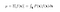The mean of a function, f, that depends on a random variable can be written as an expectation.

As mentioned earlier, if P is a simple distribution such as the uniform or normal, the mean can be computed analytically. For arbitrary distributions, P, the above computation is intractable.

## From integrals to Monte-Carlo estimates

The idea of Monte-Carlo estimates is that we replace the integral over the domain, X, with N samples of x. The average of the f computed at the samples of x approximate the true expectation. More concretely,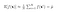Integrating over the domain of x to get the exact value of the expectation is intractable for high dimensional x. Instead we approximate this expectation by drawing samples of the random variable x, evaluating f at those samples and then taking the average. This value is called the Monte Carlo estimate of the true expectation.

We say mu hat is a Monte-Carlo estimate of mu(without the hat), the true mean . Monte-Carlo estimates are great when it’s intractable to exactly compute a function f as described earlier. The estimates (mu_hat, for example) are themselves random variables now because we evaluate f at a bunch of random variables and average them. However, an attractive property of Monte-Carlo estimates is that they are unbiased estimates, meaning expectation of the estimate is equal to the expectation of the function f: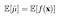Monte Carlo estimates are un-biased, meaning that expectation of these estimates is equal to the expectation of the original function.

Also, Monte-Carlo estimates have variance which is O(1/N), meaning the variance reduces with the number of samples in the order of 1/N, where N is the number of samples.

Monte-Carlo estimates are quickly roughly right but need a lot of samples to be very accurate estimates of the true expectation

# Generating samples with MCMC methods

So now we’ve seen the importance of drawing samples from a distribution and how the average of the function f computed at these samples can provide Monte-Carlo estimates of the expectation of the function. Before I go on to describing a method for drawing such independent samples from an arbitrary distribution, let’s discuss a bit about Markov chains.

## Markov Chains

A Markov Chain is a systematic way of drawing samples of a random variable, where the probability of drawing a sample at timestep t is only conditionally dependent on the the random variable drawn in the prior timestep. That is, in the example below P(x4 | x1, x2, x3) = P(x4 | x3).

The idea is that we use this Markov Chain to create a chain of samples of a random variable. But note, these samples aren’t really independent of one another because the sample at timestep t is dependent on the sample at timestep (t — 1)! Yes, but if we run this chain long enough, take all the samples from each timestep and jumble them together before we draw samples from this set, the samples drawn will asymptotically be independent (this is actually what’s called the “mixing” of a Markov Chain)

Okay, so this is where we are so far — we can approximate an expectation of a function f over a random variable x by taking a bunch of independent samples of x, evaluating f and taking its average. That’s the Monte Carlo estimate. Next, we’ve seen that we can run a Markov Chain long enough to generate independent samples of x.

But, how exactly do we create this Markov Chain? There are a few Markov Chain Monte Carlo methods (MCMC) but I’ll discuss one such method of generating a Markov Chain — Gibbs sampling!

Intuition with MH

Let’s say we want samples of x that maximize the unnormalized density T. Remember, it’s actually easy to evaluate T(x) but hard to evaluate P(x) because computing the normalization constant Z is expensive. Let’s say we sample these xs from a proposal distribution q(x’, x_t)

## Gibbs Sampling

Gibbs sampling is an MCMC technique that can help with generating independent samples from a distribution P. Let’s say we have a random variable x, which is a d-dimensional vector where each of the components x^i is an independent random variable. The idea in Gibbs sampling is to generate samples by sweeping through each dimension of x and sample from its conditional distribution with the remaining variables/dimensions fixed to their current values.

According the algorithm, we start with an intial value of the random variable x at iteration 0. These values are sampled from a prior distribution q. Then, at each iteration i, each dimension of the variable x is drawn from a conditional distribution p. We assume it’s easy to draw samples from these conditionals. It’s important to note that we aren’t drawing samples from the full joint P, which as I have discussed is hard — we are only simulating samples from this distribution P by sweeping through each of the dimensions of x at each iteration and drawing the values of each dimension from the conditional. The idea is, if we run this algorithm for enough number of iterations, then take the collect the samples of x from each iteration into a set and then draw a sample from this set, it will simulate drawing from the actual distribution P.

The animation below shows a Gibbs sampler for a bivariate Gaussian. The conditionals of a bivariate Gaussian are also Gaussians, so they’re incredibly easy to sample from! The red crosses are the samples drawn and the contour shows the actual distribution. I intentionally set the initial sample at (15, 15) but as you can see, the sampler quickly starts sampling from the higher densities. Code for this available here.